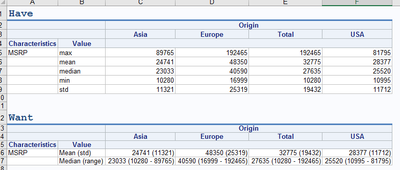## proc report - creating custom summary rows

Dear community,

I have been requested to provide a report in which mean, median, max, min and stdev are supposed to be shown in two rows: mean (std) and median(min-max) - and in this format. My existing code relies on having the results in a numeric column, which concatenated statistics are not:

``````%macro characteristics_sql_num(total=);
select "MSRP" length=100 as Characteristics,
"&summary_function" length=100 label=" " as Value,
%if %length(&total) GT 0 %then &total as;
Origin,
&summary_function(MSRP) format=5.1 as result
from sashelp.cars
%if %length(&total) EQ 0 %then group by Origin;
%mend characteristics_sql_num;

%macro characteristics_num;
%let summary_functions = mean std min median max;

proc sql;
create table cars_summary as

%do i = 1 %to %sysfunc(countw(&summary_functions));
%let summary_function = %scan(&summary_functions, &i);

%if &i NE 1 %then
union;

%characteristics_sql_num
union
%characteristics_sql_num(total="Total")
%end;
;
%mend characteristics_num;

%characteristics_num

proc report data=cars_summary;
columns Characteristics Value Origin, result;
define Characteristics -- Value / group;
define Origin / across;
define result / " " format=best6.;
run;``````

I tried inserting the needed rows with break after and compute after, but this unfortunately does not work. Is there a way of generating the needed rows during proc report, or should I rather rebuild my macro to generate the needed concatenations already during proc sql? Thank you for your support in advance.

1 ACCEPTED SOLUTION

Accepted Solutions

## Re: proc report - creating custom summary rows

Going with your Proc SQL approach, I would combine the stats already in the Proc SQL into a single var so Proc REPORT will just do the display.

Here is an example

``````
proc sql;
create table result as
select
"MSRP" as characteristic
, origin
, "mean (std)" as value
, cats(mean(msrp), "(", std(msrp), ")") as cell
from
sashelp.cars
group by
origin

union

select
"MSRP" as characteristic
, origin
, "median (range)" as value
, cats(median(msrp), "(", catx(" ", min(msrp), max(msrp)), ")") as cell
from
sashelp.cars
group by
origin
union
select
"MSRP" as characteristic
, "Total"
, "mean (std)" as value
, cats(mean(msrp), "(", std(msrp), ")") as cell
from
sashelp.cars

union

select
"MSRP" as characteristic
, "Total"
, "median (range)" as value
, cats(median(msrp), "(", catx(" ", min(msrp), max(msrp)), ")") as cell
from
sashelp.cars
;
quit;

proc report data=result;
column characteristic value Origin, cell;
define characteristic / group ;
define value / group ;
define origin / across ;
define cell / group " " style={textalign=right};
run;``````
3 REPLIES 3

## Re: proc report - creating custom summary rows

Not knowing how exactly the output needs to look (an example would help very much). Have a look at Proc TABULATE, it allows to have statistics on the row level.

See example below

``````
proc tabulate data=sashelp.cars format=comma14.2;
class origin;
var msrp;
table
msrp="stats 1"*(mean std)
msrp="stats 2"*(min median max)
,
origin=" " all
;
run;``````

## Re: proc report - creating custom summary rows

Thanks for responding! Switching to proc tabulate would unfortunately mean rewriting much of the available macros so I am afraid it is not feasible. It would probably be easier to adapt proc sql statements. Below is what I have vs what has been requested:As I am using ODS excel, maybe there could be a way of putting in a formula which generates the needed format instead of handling it all in proc report?

## Re: proc report - creating custom summary rows

Going with your Proc SQL approach, I would combine the stats already in the Proc SQL into a single var so Proc REPORT will just do the display.

Here is an example

``````
proc sql;
create table result as
select
"MSRP" as characteristic
, origin
, "mean (std)" as value
, cats(mean(msrp), "(", std(msrp), ")") as cell
from
sashelp.cars
group by
origin

union

select
"MSRP" as characteristic
, origin
, "median (range)" as value
, cats(median(msrp), "(", catx(" ", min(msrp), max(msrp)), ")") as cell
from
sashelp.cars
group by
origin
union
select
"MSRP" as characteristic
, "Total"
, "mean (std)" as value
, cats(mean(msrp), "(", std(msrp), ")") as cell
from
sashelp.cars

union

select
"MSRP" as characteristic
, "Total"
, "median (range)" as value
, cats(median(msrp), "(", catx(" ", min(msrp), max(msrp)), ")") as cell
from
sashelp.cars
;
quit;

proc report data=result;
column characteristic value Origin, cell;
define characteristic / group ;
define value / group ;
define origin / across ;
define cell / group " " style={textalign=right};
run;``````
Discussion stats
• 3 replies
• 444 views
• 0 likes
• 2 in conversation